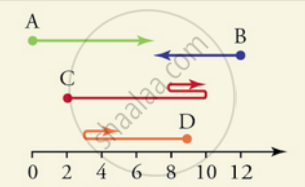Share

# Distance and Displacement

Course

#### notes

Distance: The distance covered by an object is described as the total path length covered by an object between two endpoints.

Distance is a numerical quantity. We do not mention the direction in which an object is travelling while mentioning about the distance covered by that object.

Displacement: The shortest possible distance between the initial and final position of an object is called Displacement. Displacement is denoted by Δx.

Zero Displacement: When the first and last positions of an object are same, the displacement is zero.

Negative Displacement and Positive Displacement:Here, displacement of object B is negative

ΔB = Bf − B0

= 7–12

= –5

Also, displacement of object A is positive

ΔA = Af − A0

= 7– 0

= 7

 Distance Displacement It is the actual path length travelled by an object. It is the shortest distance between initial point and final point. It is a scalar quantity. It is a vector quantity,. It is always positive and cant be zero. It can be positive, negative or zero

Vectors and Scalar Quantities:

• Vector is a quantity which have both magnitude and direction. For examples: Force, position etc.
• Scalar is quantity with which direction is not associated. For examples: Mass, temperature, time etc.

### Shaalaa.com

Motion part 3 (Scalar and vector quantity) [00:04:41]
S
series 1
0%

S